## Helmholtz Differential Equation--Cartesian Coordinates

In 2-D Cartesian Coordinates, attempt Separation of Variables by writing(1)

then the Helmholtz Differential Equation becomes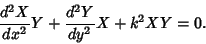(2)

Dividing both sides bygives(3)

This leads to the two coupled ordinary differential equations with a separation constant,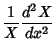(4)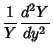(5)

whereand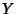could be interchanged depending on the boundary conditions. These have solutions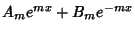(6)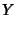(7)

The general solution is then(8)

In 3-D Cartesian Coordinates, attempt Separation of Variables by writing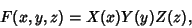(9)

then the Helmholtz Differential Equation becomes(10)

Dividing both sides bygives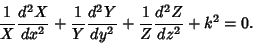(11)

This leads to the three coupled differential equations(12)(13)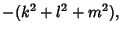(14)

where,, andcould be permuted depending on boundary conditions. The general solution is therefore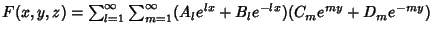(15)

References

Morse, P. M. and Feshbach, H. Methods of Theoretical Physics, Part I. New York: McGraw-Hill, pp. 501-502, 513-514 and 656, 1953.